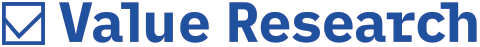# How is RD interest calculated?

##### The Recurring Deposit (RD) interest is compounded quarterly

How is interest calculated on RDs? Is there a formula to know how much interest is earned on RDs after four years, for a 5 year tenure?
- Rajkumar Patange

The Recurring Deposit (RD) interest is compounded quarterly. And the formula to calculate the RD returns is the same as the formula for compound interest.

Say, for example, you invested ₹1000 per month for 5 years in a RD account. Assuming a rate of interest of 8 percent per annum, the maturity value of the same would be ₹73862.